### Home > CAAC > Chapter 2 > Lesson 2.1.6 > Problem2-63

2-63.
1. Decide if each of the statements below is sometimes true, always true, or never true. Justify your conclusion. If the statement is not always true, produce a counterexample (an example that shows the statement can or must be false) to help support your claim. Homework Help ✎

1. If y = 3, then 2y = 6.

2. If x + 3 = 9, then x = 2.

3. If the product of two numbers is positive (meaning more than zero), then both numbers must be positive.

4. If a + b = b, then a = 0.

5. If a and b are both odd integers, then ab is even.

6. If x is greater than zero, then −x is less than zero.

7. If a is prime (meaning that a is an integer greater than 1 and has no other factors besides 1 and itself), then (a + 1) is not prime.

8. If x is greater than y, and both x and y are not zero, then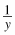is greater than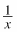.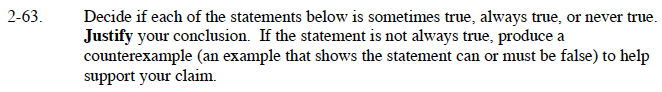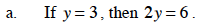Substitute the y-value into the equation

2(3) = 6

6 = 6
Always true because if a = b, then ac = bc.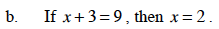Substitute the x value into the equation.

2 + 3 = 9

Never true, because 2 + 3 ≠ 9 since 5 ≠ 9.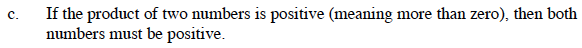Is there another way to create a positive product, without using positive numbers?

Sometimes true, because a (positive) · (positive) = (positive) but a (negative) · (negative) = (positive) also.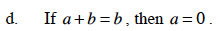Review the properties of equality studied earlier in this chapter.

Always true, because according to the Identity Property of Addition 0 + b = b so a = 0.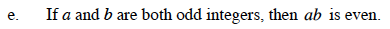Try a few examples to see if a pattern emerges.

(3)(7) = ?
(5)(11) = ?
(7)(11) = ?

Never true, because the product of two odds is always odd.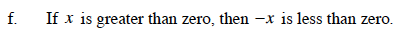Always true, because if x is positive −x will always be the opposite which is negative.
So the answer will be less than zero.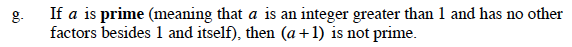Try a few examples of prime numbers starting with 2.

2 + 1 = ?
3 + 1 = ?
5 + 1 = ?
7 + 1 = ?
What do you notice?

Sometimes true, because there are instances where the sum is prime(when a = 2) and instances where the sum is not prime.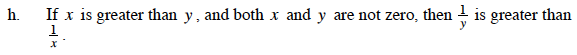Make sure to try values of x and y that are positive and negative, where x > y.

Are these situations true or false?

x = 3 and y = 2 so, x > y, then

$\frac{1}{2}>\frac{1}{3}$

x = −2 and y = −5, so x > y, then

$-\frac{1}{5}>-\frac{1}{2}$

Always true.